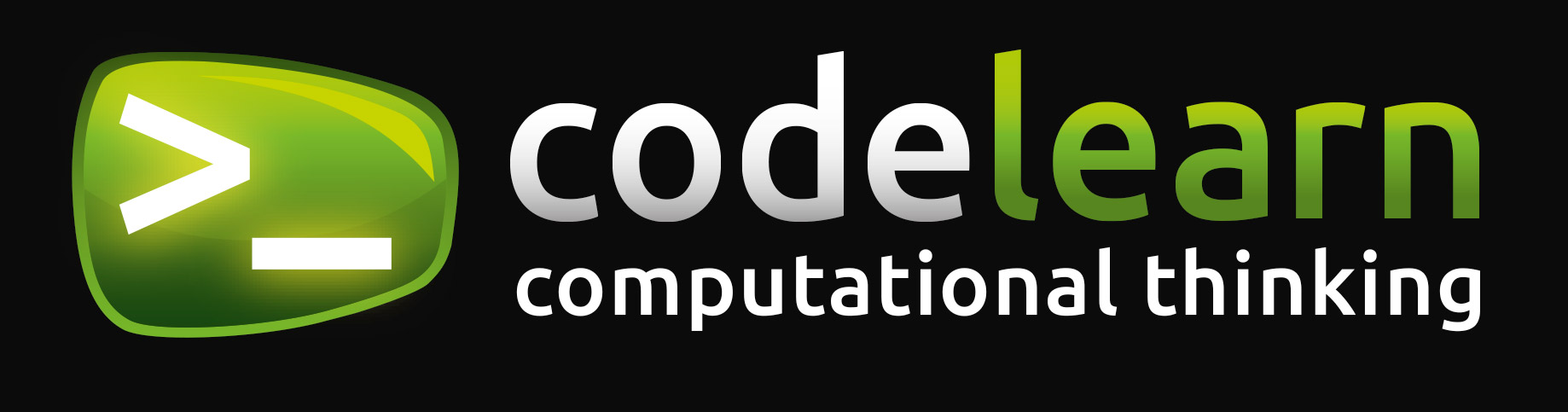Coming soon

This too :)
info@codelearn.com

Do you know how to count to 31 with one hand?

Do you know how to count to 31 with one hand?

What is the binary system?

The binary system is a numbering system in which numbers are represented using only two digits: zero (0) and one (1). It is the natural counting system used by computers, since internally they work with two levels of voltage: on (or with presence of electric charge, equivalent to 1) and off (or without electric charge, equivalent to 0).

Brief historical introduction

The first known description of the binary system goes back to the third century before our era, when the indian mathematician Pingala discovered the concept of the number zero. For example, later uses of the binary system can be found in classical texts of ancient China or in some treatises of Sir Francis Bacon (one of the fathers of the current science) in which he spoke of using the binary system to encode the alphabet.

The modern binary system was documented in its entirety by Leibniz in the seventeenth century, in his article “Explication de l’Arithmétique Binaire”. In this, Leibniz already used 0 and 1, as in the current binary numbering system.

It was not until 1854 that the binary system gained the notoriety it now possesses: the British mathematician George Boole published an article detailing a system of logic (which would eventually be called Boolean Algebra) based on the theory of the binary system. Boole’s Algebra became a fundamental factor for the development of current electronic systems.

Representation of a decimal number in a binary system

The number in decimal system is divided by 2. The result of this division is re-divided by 2, and so on until the dividend is smaller than the divisor (2). That is, when the number to be divided is 1, the division is finished. Subsequently, the residues of the division are sorted from the last to the first (they are placed in the reverse order of how they appear in the divisions). This will be the binary number resulting from the conversion.

Counting in binary system with the fingers of the hand

To count in binary system using the fingers of one hand it is necessary, initially, to know some more characteristics of the binary system:

• Depending on the number of digits we use to represent our numbers in binari we can represent more or less decimal numbers. For example, if we only use one digit for the binary representation, we can only represent two decimal numbers: 0 and 1. If we use two digits for our binary system we can represent four decimal numbers: 0, 1, 2 i 3. If we use three digits, we can represent eight decimal numbers: 0, 1, 2, 3, 4, 5, 6 and 7. I so on. The relation says that being n the number of binary digits to use, we will be able to represent 2n – 1 (including 0).
• The digits of a number in binary system (in the world of computer science) are also known as bits.
• Each bit of a number in binary system is assigned a weight depending on its position. Thus, the rightmost digit is the highest weight digit and the leftmost digit is the lowest weight digit.
• The weight of the digits indicates the value that a certain digit contributes with to the calculation of its decimal equivalent when it is active. We always start counting from the lowest weight bit to the highest weight bit. Thus, the lower weight bit (the leftmost bit) gives a value of 0 if it is not active and a value of 20 if it is active (1). The second bit of least weight gives a value of 0 if it is not active and a value of 21 if it is active (2). The third bit of less weight adds a value of 0 if it is not active and a value of 22 if it is active (4). I so on. The general rule says that given a bit in the position n its contribution is 2n-1 when it is active, and 0 otherwise.

¡Knowing this, we are already prepared to learn to count in binary with our hands! The process consists in raising the right hand and putting it with the closed fist facing toward us. Seen like this, our hand consists of five bits, where the weightless bit belongs to the thumb, and the weight most bit to that of the little finger. We will say that a bit is active if the finger is raised. We will say that a bit is inactive if the finger is down. How many decimal numbers can we represent with a hand? According to what we have explained previously, if we have 5 digits we can represent the decimals up to 25 – 1, that is, (2 x 2 x 2 x 2 x 2) – 1 = 32 – 1 = 31 decimal numbers and 0 (which is the absence of representation).

Don’t you believe it? Look!

And this using one single hand! What about using both hands? Do you believe it if we tell you that you can be count until 1023? Give it a try! Power is in your hands!